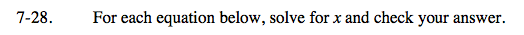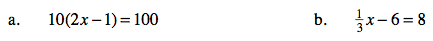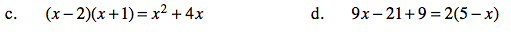Home > CAAC > Chapter 7 > Lesson 7.1.3 > Problem7-28

7-28.
1. For each equation below, solve for x and check your answer. Homework Help ✎

1. 10(2x − 1) = 100

2.− 6 = 8

3. (x − 2)(x + 1) = x2 + 4x

4. 9x − 21 + 9 = 2(5 − x)For more help solving equations, see problem 6-107.5.5

42